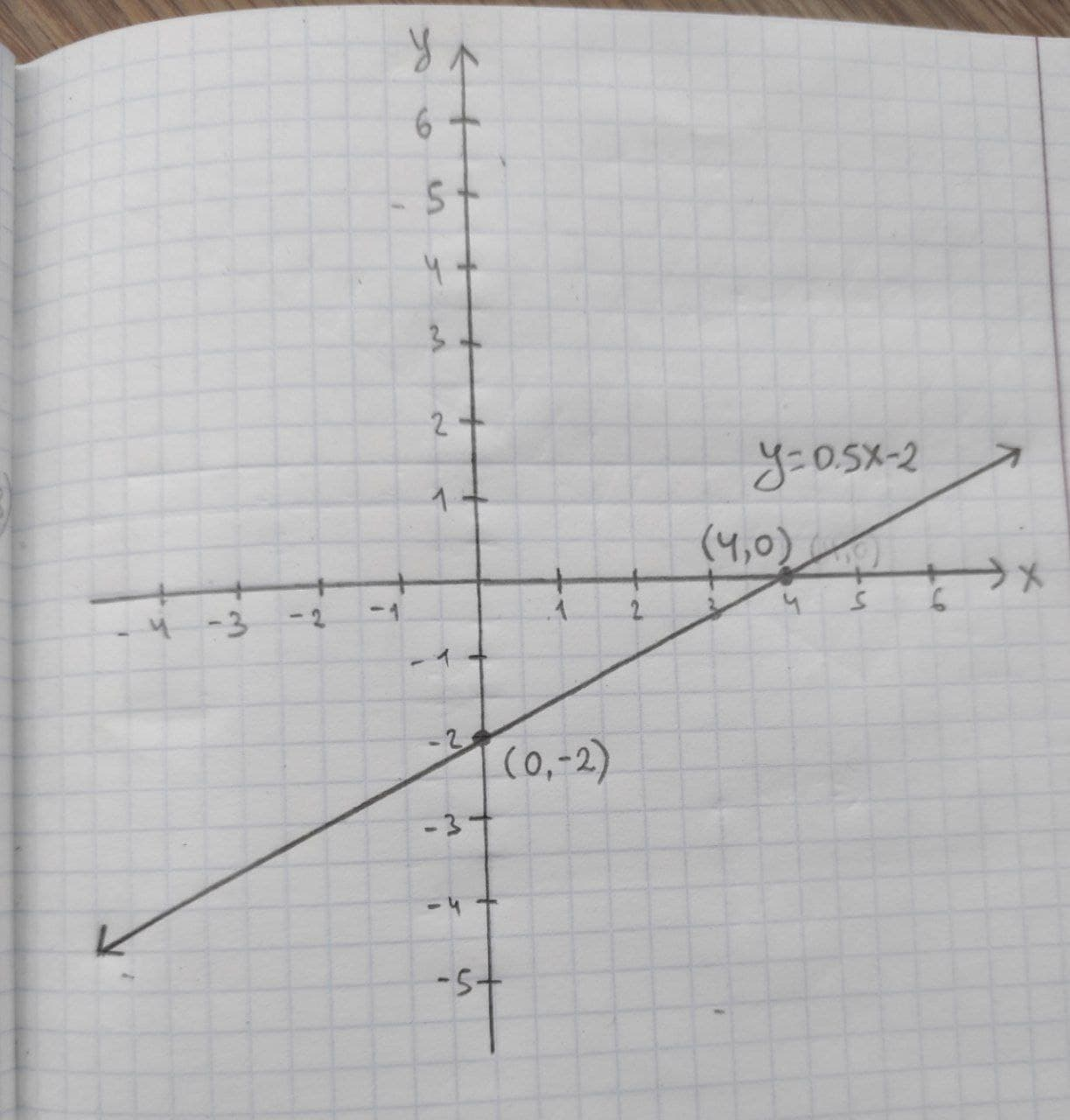necessaryh

2020-11-05

Given linear equation y = 0.5x – 2
a. find the y-intercept and slope.
b. determine whether the line slopes upward, slopes downward, or is horizontal, without graphing the equation.
c. use two points to graph the equation.casincal

Expert

(a) y-intercept and slope: In a linear equation $y={b}_{0}+{b}_{1}x$, the constant $b1$ be the slope and ${b}_{0}$ be the y-intercept form and x is the independent variable and y is the dependent variable. Comparing the given equation with the general form of linear equation the slope of the equation is 0.5 and the y-intercept is –2. Therefore, the y-intercept is –2 and the slope of the linear equation (b_1) is 0.5. (b) It is known that, the slope of the linear equation $y={b}_{0}+{b}_{1}x$ is upward if b_1 > 0, the slope of the linear equation $y={b}_{0}+{b}_{1}x$ is downward if ${b}_{1}<0$, and the slope of the linear equation $y={b}_{0}+{b}_{1}x$ is horizontal if ${b}_{1}=0$ .
Thus, in the given equation $y=0.5x–2,{b}_{1}$ is 0.5, which is greater than 0.
Thus, the slope is upward.
(c) Graph by using two points:
The two points $\left({x}_{1},{y}_{1}\right)\phantom{\rule{1em}{0ex}}\text{and}\phantom{\rule{1em}{0ex}}\left({x}_{2},{y}_{2}\right)$ on the given line are obtained as follows:
If x=0
$y=\left(0,5×0\right)-2$
y=-2
Thus, one point on the line is $\left({x}_{1},{y}_{1}\right)=\left(0,-2\right)$
If x=4,
$y=\left(0.5×4\right)-2$
y=0
Thus, the second point on the line is $\left({x}_{2},{y}_{2}\right)=\left(4,0\right)$Do you have a similar question?